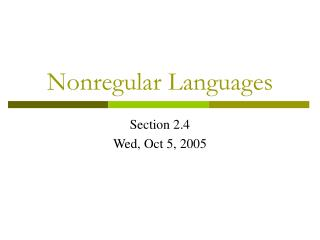Download PresentationNonregular Languages# Nonregular Languages - PowerPoint PPT Presentation

Download Presentation##### Nonregular Languages

Download Policy: Content on the Website is provided to you AS IS for your information and personal use and may not be sold / licensed / shared on other websites without getting consent from its author. While downloading, if for some reason you are not able to download a presentation, the publisher may have deleted the file from their server.

- - - - - - - - - - - - - - - - - - - - - - - - - - - E N D - - - - - - - - - - - - - - - - - - - - - - - - - - -
##### Presentation Transcript

1. Nonregular Languages Section 2.4 Wed, Oct 5, 2005

2. Countability of the Set of DFAs • Theorem: The set of all DFAs (over an alphabet ) is countable. • Proof: • For a given n > 0, let Sn be the set of all DFAs with exactly n states. • How many DFAs are in Sn? • There are n choices for the initial state. • For each state, there are n|| choices for the transitions coming out of that state. • Therefore, there are (n||)n = n||n choices for .

3. Countability of the Set of DFAs • There are 2n choices for the final states. • Therefore, the number of DFAs with exactly n states is nn||n 2n. • The set of all DFAs is S1S2S3 … • This is a countable set since it is the union of a countable number of finite sets. • Thus, we can enumerate the DFAs as M0, M1, M2, M3, …

4. The Existence of a Non-Regular Language • There exists a language that is not accepted by any DFA (provided  ). • Proof: • Let Ln = L(Mn). • Let x be any symbol in . • Let sn = xn, for all n 0. • Define a new language L by the rule that snL if and only if snLn. • Then Lis not equal to any Ln. • So L is not accepted by any DFA.

5. The Existence of a Non-Regular Language • This is another example of a diagonalization argument. • It is a non-constructive proof. • It does not provide us with an example (unless we actually figure out what each Mn is!).

6. The Existence of a Non-Regular Language • Another non-constructive proof is based on a cardinality argument. • The set of all languages is 2*, which is uncountable since its cardinality is equal to the cardinality of 2N, which we know to be uncountably infinite. • The set of DFAs is countable. • Therefore, the function f(M) = L(M) cannot be onto2*. • So, what is an example of a nonregular language?

7. The Pumping Lemma • The Pumping Lemma: Let L be an infinite regular language. There exists an integer n 1 such that any string wL, with |w| n, can be represented as the concatenation xyz such that • y is non-empty, • |xy| n, and • xyizL for every i 0.

8. Proof of the Pumping Lemma • Proof: • Let n be the number of states. • Let w be any string in L with at least n symbols. • After processing n symbols, we must have returned to a previously visited state (the Pigeonhole Principle). • Let q be the first revisited state. • Let x be the string processed from s to q. • Let y be the string processed around the loop from q back to q. • Let z be the string from q to the end, a final state f.

9. Proof, continued • Then clearly |y| > 0 and |xy| n. • It is also clear that xyizL for all i 0, since we may travel the loop as many times as we like, including 0 times.

10. The Pumping Lemma • The Pumping Lemma says that if L is regular, then certain properties hold. • The contrapositive of the Pumping Lemma says that if certain properties do not hold, then L is not regular. • Therefore, you cannot use the Pumping Lemma to conclude that a language is regular, but only that it is not regular. • That’s good, because that is exactly what we want to do.

11. The Standard Example of a Nonregular Language • Let L = {aibi | i 0}. • Suppose that L is regular. • “Let n be the n of the Pumping Lemma” and consider the string w = anbn. • Then w can be decomposed as xyz where |y| > 0 and |xy| n. • Therefore, xy consists only of a’s. • It follows that y = ak, for some k > 0.

12. Standard Example of a Nonregular Language • According to the Pumping Lemma, xy2z is in L. • However, xy2z =an + kbn, which is not in L. since n + kn. • This is a contradiction. • Therefore, L is not regular.

13. A Second Example of a Nonregular Language • Let L = {w *| w contains an equal number of a’s and b’s}. • Suppose L is regular. • Let L1 = L(a*b*). • Then LL1 = {aibi | i 0} would also be regular, which is a contradiction. • Therefore, L is not regular.

14. More Examples • {w *| w contains an unequal number of a’s and b’s}. • {w *| w contains more a’s than b’s}. • {w * | w = zz for some z  *}. • Consider w = anbanb and use the Pumping Lemma. • {w * | w  zz for some z  *}. • Notice that we use the Pumping Lemma only when necessary; other arguments are often simpler.